﻿ 基于协同进化算法的支持向量回归径流建模研究与应用 Evolving Support Vector Regression by Co-Evolution Algorithm for Runoff Forecasting Modeling Research and Application

Computer Science and Application
Vol. 10  No. 03 ( 2020 ), Article ID: 34620 , 16 pages
10.12677/CSA.2020.103053

Evolving Support Vector Regression by Co-Evolution Algorithm for Runoff Forecasting Modeling Research and Application

Jiansheng Wu1, Yongsheng Xie1, Long Jin2

1Department of Mathematical and Computer Sciences, Guangxi Science & Technology Normal University, Liuzhou Guangxi

2Guangxi Climate Center, Nanning Guangxi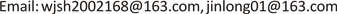Received: Feb. 29th, 2020; accepted: Mar. 13th, 2020; published: Mar. 20th, 2020ABSTRACT

Aiming at the difficulty of selecting the appropriate kernel function type, kernel function parameters, penalty coefficients of support vectors, and insensitive loss parameters in practical applications of support vector model, in this paper, co-evolution algorithm is employed to simultaneously evolve selection the data features, the kernel type of support vector regression, the parameters of the kernel function and the model parameters, namely HPSOSA-SVR by hybrid the strengths of Particle Swarm Optimization (PSO) and Simulated Annealing (SA). In order to select the optimal support vector regression kernel function type, the optimal matching kernel function parameter and the support vector model parameter for runoff modelling, the co-evolution algorithm focuses on combining the advantages of PSO (fast calculation and easy mechanism) and SA (ability to jump away from local optimum solutions and converge to the global optimum solution) by combined the metropolis processes of SA into the movement mechanism and parallel processing of PSO. The HPSOSA algorithm has the capability of both fast calculation and searching in the direction of the global optimum solution, helping PSO jump out of local optima, avoiding into the local optimal solution early and leads to a good solution. Compared with HPSOSA, PSO and pure SVR, results show that the HPSOSA-SVR model can successfully identify the optimal type of kernel function and all the optimal values of the parameters of SVR with the lowest prediction error values in runoff forecasting, can significantly improve the runoff forecasting accuracy. Experimental results reveal that the predictions using the proposed approach are consistently better than those obtained using the other methods presented in this study in terms of the same measurements.

Keywords:Particle Swarm Optimization, Simulated Annealing Algorithm, Support Vector Regression, Kernel Function, Runoff Modeling1广西科技师范学院数学与计算机科学学院，广西 柳州

2广西气象局，广西 南宁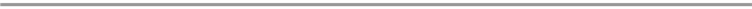1. 引言

20世纪90年代由Vapnik等人提出的统计学习理论，在此基础上发展起来的支持向量机是一种新型机器学习方法。它是基于结构风险最小化原则，集成了最大间隔超平面、核技术、凸二次规划、稀疏解和松弛变量等多项技术  ，在解决小样本、非线性及高维空间学习时显示了其卓越的优越性，被成功应用到函数拟合逼近，文本和手写识别等领域    。SVM在实际应用建模过程中，但是对于建模数据的特征选择、支持向量的核函数类型、核函数的参数以及支持向量的惩罚系数和不敏感损失函数参数没有理论指导，实际应用中采用交叉验证方法确定参数。这种方法虽然简单，但是过多地依赖设计者的经验，缺乏理论基础，得到的结果不稳定，这些问题影响SVM模型在实际应用中推广    。

2. 混合进化支持向量回归建模

2.1. 支持向量机

$f\left(x\right)={\omega }^{\text{T}}\phi \left(x\right)+b$ (1)

$\left\{\begin{array}{l}\mathrm{min}\left(\omega ,\xi ,{\xi }^{*}\right)=\frac{1}{2}{\omega }^{\text{T}}\omega +C{\sum }_{i=1}^{N}\left(\xi +{\xi }^{*}\right)\\ s.b.\text{\hspace{0.17em}}\text{\hspace{0.17em}}{y}_{i}-{\omega }^{\text{T}}\phi \left({x}_{i}\right)-b\le \epsilon +\xi \\ \text{\hspace{0.17em}}\text{\hspace{0.17em}}\text{\hspace{0.17em}}\text{\hspace{0.17em}}\text{\hspace{0.17em}}\text{\hspace{0.17em}}\text{\hspace{0.17em}}-{y}_{i}+{\omega }^{\text{T}}\phi \left({x}_{i}\right)+b\le \epsilon +{\xi }^{*}\\ \text{\hspace{0.17em}}\text{\hspace{0.17em}}\text{\hspace{0.17em}}\text{\hspace{0.17em}}\text{\hspace{0.17em}}\text{\hspace{0.17em}}\xi ,{\xi }^{*}\ge 0,i=1,2,\cdots ,N\end{array}$ (2)

$f\left(x\right)={\sum }_{i=1}^{N}\left({\beta }_{i}^{*}-{\beta }_{i}\right)K\left({x}_{i},x\right)+b$ (3)

$K\left({x}_{i},{x}_{j}\right)=\phi \left({x}_{j}\right)\circ \phi \left({x}_{i}^{\text{T}}\right)$ (4)

${K}_{1}\left({x}_{i},{x}_{j}\right)={x}_{i}{x}_{j}^{\text{T}}$ (5)

${K}_{2}\left({x}_{i},{x}_{j}\right)={\left(t+c{x}_{i}{x}_{j}^{\text{T}}\right)}^{d}$ (6)

${K}_{3}\left({x}_{i},{x}_{j}\right)=\mathrm{exp}\left(-\frac{{‖{x}_{i}-{x}_{j}‖}^{2}}{2{\sigma }^{2}}\right)$ (7)

${K}_{4}\left({x}_{i},{x}_{j}\right)=\mathrm{tanh}\left[\alpha \left({x}_{i}{x}_{j}^{\text{T}}+\beta \right)\right]$ (8)

SVR的性能主要取决于三个因素：1) 核函数的类型及其核函数参数；2) SVR模型中惩罚系数 和不敏感损失函数的参数 $\epsilon$ ；3) 建模数据集的特征信息。首先核函数类型的选择是决定的SVR模型的主要因素，核函数是反映数据的先验知识，决定训练数据高维特征空间情况，不恰当的核函数会使得训练样本在高维空间的分布变得比输入空间的分布更复杂，核函数的构造对于特定的核方法固然重要，但当核函数构造完毕(核函数的类型固定)后，其次核函数中的参数最优匹配同等重要。研究表明，针对同一个核函数，选择不同的核函数的参数，SVR的泛化性能会相差很大 ，这主要是因为不同的参数对应的高维特征空间的结构具有差异性，而特征空间的性质直接决定着核方法的性能。再次SVR模型的惩罚系数和不敏感损失函数参数也是两个重要参数，其中惩罚系数控制着最小训练误差和最小化模型复杂度之间的平衡，目的是在总体误差与学习机模型复杂性之间进行折衷，即在确定的特征空间中调节经验风险和学习机置信范围的比例，以使得学习机器的泛化能力最好，而不敏感损失函数参数是度量训练点上误差的代价，在模型的光滑性和泛化性之间起着平衡作用    。最后建模数据最优特征信息选择对SVR模型性能有一定的影响，由于建模因子数据在收集中包含有噪声，维数高，而且数据是多数据源或异构数据集，如果不对原始建模因子数据做特征提取处理，将会影响SVR的性能，增加模型的复杂度影响模型泛化性能    。

2.2. 粒子群优化和模拟退火算法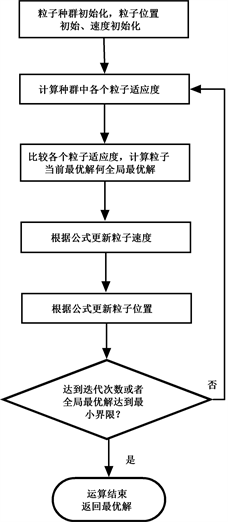Figure 1. Flow chart of PSO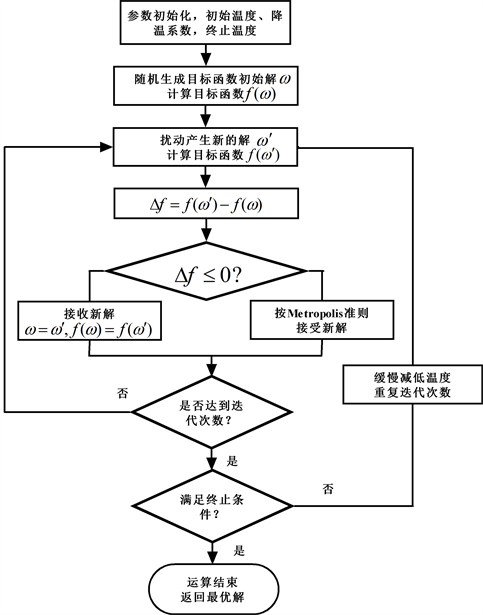Figure 2. Flow chart of SA

2.3. 粒子群–模拟退火算法协同进化支持向量回归建模

$f\left(T\right)=\frac{1}{{N}_{1}}{\sum }_{i=1}^{{N}_{1}}{\left({y}_{i}-{\stackrel{^}{y}}_{i}\right)}^{2}$ (9)

$\left\{\begin{array}{l}\mathrm{max}f\left(T\right)=\frac{1}{{N}_{1}}{\sum }_{i=1}^{{N}_{1}}{\left({y}_{i}-{\stackrel{^}{y}}_{i}\right)}^{2}\\ s.b.\text{\hspace{0.17em}}\text{\hspace{0.17em}}{\stackrel{^}{y}}_{i}={\sum }_{i=1}^{{N}_{1}}\left({\beta }_{i}^{\text{*}}-{\beta }_{i}\right)K\left({c}_{i}{x}_{i},x\right)+b\\ \text{\hspace{0.17em}}\text{\hspace{0.17em}}\text{\hspace{0.17em}}\text{\hspace{0.17em}}\text{\hspace{0.17em}}\text{\hspace{0.17em}}\text{\hspace{0.17em}}\left\{\begin{array}{l}\mathrm{min}\left(\omega ,\xi ,{\xi }^{\text{*}}\right)=\frac{1}{2}{\omega }^{\text{T}}\omega +C{\sum }_{i=1}^{N}\left(\xi +{\xi }^{\text{*}}\right)\\ s\text{.}b\text{.}\text{\hspace{0.17em}}\text{\hspace{0.17em}}{y}_{i}-{\omega }^{\text{T}}\phi \left({x}_{i}\right)-b\le \epsilon +\xi \\ \text{\hspace{0.17em}}\text{\hspace{0.17em}}\text{\hspace{0.17em}}\text{\hspace{0.17em}}\text{\hspace{0.17em}}\text{\hspace{0.17em}}\text{\hspace{0.17em}}-{y}_{i}+{\omega }^{\text{T}}\phi \left({x}_{i}\right)+b\le \epsilon +{\xi }^{\text{*}}\\ \text{\hspace{0.17em}}\text{\hspace{0.17em}}\text{\hspace{0.17em}}\text{\hspace{0.17em}}\text{\hspace{0.17em}}\text{\hspace{0.17em}}\text{\hspace{0.17em}}\xi ,{\xi }^{\text{*}}\ge 0,i=1,2,\cdots ,N\end{array}\\ \text{\hspace{0.17em}}\text{\hspace{0.17em}}\text{\hspace{0.17em}}\text{\hspace{0.17em}}\text{\hspace{0.17em}}\text{\hspace{0.17em}}\text{\hspace{0.17em}}\text{\hspace{0.17em}}{c}_{i}\in \left\{0,1\right\},i=1,2,\cdots {N}_{1}\\ \text{\hspace{0.17em}}\text{\hspace{0.17em}}\text{\hspace{0.17em}}\text{\hspace{0.17em}}\text{\hspace{0.17em}}\text{\hspace{0.17em}}\text{\hspace{0.17em}}\text{\hspace{0.17em}}{K}_{j}\in \left\{{K}_{1},{K}_{2},{K}_{3},{K}_{4}\right\}\end{array}$ (10)Table 1. Relevant parameter coding components involved in the SVR model

1) 参数初始化，随机生成n行 ${m}_{1}$ 列布尔代数矩阵和n行 ${m}_{2}$ 列浮点数矩阵，n表示种群个数，本文n = 40，粒子群种群个数40个， ${m}_{1}$ 是影响径流变量建模因子个数和四个核函数决定，为此 ${m}_{1}=N+4$${m}_{2}$ 是核函数参数个数和模型参数决定，故此 ${m}_{2}=4$。布尔代数是控制矩阵，控制变量选择和核函数类型，浮点数矩阵取上的 $\left[0,10\right]$ 均匀分布随机数，它控制核函数的参数和SVR模型参数的大小。

2) 计算适应度，输入训练样本并标准化，通过解码每行布尔代数矩阵和浮点数矩阵，即可获得参与计算建模样本因子、核函数类型、核函数参数和模型参数，输入相应选择建模样本的特征，通过(10)式计算每个粒子的适应度，比较种群个体的适应度获得最好位置 ${p}_{best}\left(t\right)$，以及群体中全局的最好位置 ${p}_{gest}\left(t\right)$

3) 粒子速度更新方程为：

${v}_{i}^{t+1}=\omega {v}_{i}^{t}+{c}_{1}{r}_{1}\left({p}_{best}\left(t\right)-{s}_{i}^{t}\right)+{c}_{2}{r}_{2}\left({p}_{gbest}\left(t\right)-{s}_{i}^{t}\right)$ (11)

$\omega ={\omega }_{\mathrm{max}}-\frac{{\omega }_{\mathrm{max}}-{\omega }_{\mathrm{min}}}{ite{r}_{\mathrm{max}}}\cdot iter$ (12)

4) 粒子个体位置更新分为二进制离散粒子群和实数粒子群的更新，二进制离散粒子群位置更新方程为：

${s}_{i}^{t+1}=\left\{\begin{array}{l}0,\text{\hspace{0.17em}}\text{\hspace{0.17em}}\text{\hspace{0.17em}}r\ge \frac{1}{1+\mathrm{exp}\left(-{v}_{i}^{t+1}\right)}\\ 1,\text{\hspace{0.17em}}\text{\hspace{0.17em}}\text{\hspace{0.17em}}r<\frac{1}{1+\mathrm{exp}\left(-{v}_{i}^{t+1}\right)}\end{array}$ (13)

${s}_{i}^{t+1}={s}_{i}^{t}+{v}_{i}^{t+1}$ (14)

5) 计算新的位置和旧的位置适应度之差 $\Delta f=f\left({s}_{i}^{t+1}\right)-f\left({s}_{i}^{t}\right)$，若适应度变大，则新的位置被接受，作为下一代的粒子，若适应度变小，对其进行模拟退火Metropolis抽样准则接收进入下一代。在 $\Delta f<0$ 时，在 $\left[0,\text{1}\right]$ 均匀分布生成的随机数R，在当前温度 ${T}_{i}$ 下，如果 $R>{\text{e}}^{\left(\Delta f/{T}_{i}\right)}$，则接收到下一代 ${s}_{i}^{t+1}$，否则，在当前温度 ${T}_{i}$ 下，对其粒子速度高斯扰动，产生新的速度，并以此更新位置，使其达到抽样稳定准则。

6) 反复进行2)~5)，直到适应度满足要求或者达到总的进化代数(总的进化代数)。

7) 把进化后的最后一代适应度最高个体解码，得到相应SVR模型建模样本的特征、核函数的类型、核函数的参数和模型参数，输入测试样本进行预测，HPSOSA-SVR算法的流程框架如图3所示：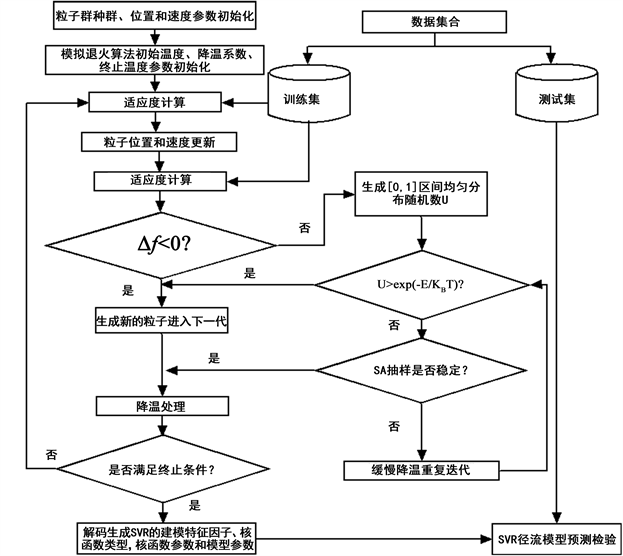Figure 3. Flow chart of the HPSOSA-SVR algorithm

3. 应用实例及其结果分析

3.1. 建模数据Table 2. Establish model training data and test model test data

3.2. 建模因子

3.3. 模型参数和性能评价

1) 均方根误差(Root Mean Square Error, RMSE)：

$RMSE=\sqrt{\frac{1}{n}{\sum }_{t=1}^{n}{\left({y}_{t}-{\stackrel{^}{y}}_{t}\right)}^{2}}$ (15)

2) 平均绝对百分比误差(Mean Absolute Percentage Error, MAPE)：

$MAPE=\frac{100}{n}{\sum }_{t=1}^{n}\frac{|{y}_{t}-{\stackrel{^}{y}}_{t}|}{|{y}_{t}|}$ (16)

3) 纳什效率系数(Nash-Sutcliffe Efficiency coefficient, NSE)：

$SE=1-\frac{{\sum }_{t=1}^{n}{\left({y}_{t}-{\stackrel{^}{y}}_{t}\right)}^{2}}{{\sum }_{t=1}^{n}{\left({y}_{t}-\stackrel{^}{y}\right)}^{2}}$ (17)

4) 峰值纳什效率系数

$NS{E}_{peak}=1-\frac{{\sum }_{i=1}^{p}{\left({y}_{pi}-{\stackrel{^}{y}}_{pi}\right)}^{2}}{{\sum }_{i=1}^{p}{\left({y}_{pi}-{\stackrel{^}{y}}_{pi}\right)}^{2}}$ (18)

3.4. 结果分析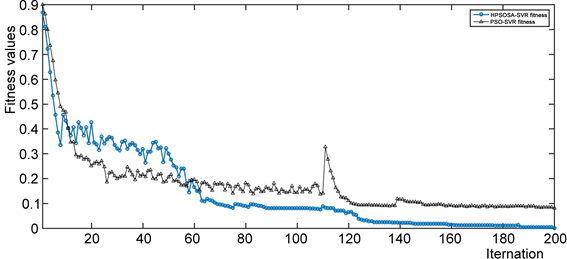Figure 4. Fitness values of PSO-SVR and HPSOSA-SVR process with the iterations

Table 3. Fitting and prediction results of three models during the 2008 flood season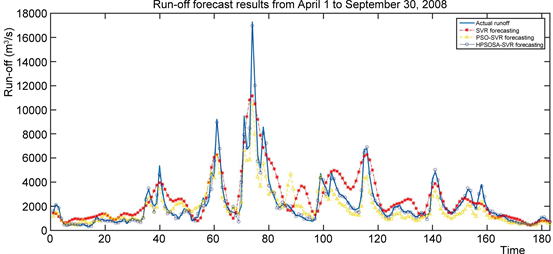Figure 5. Prediction results of runoff from April 1 to September 30, 2008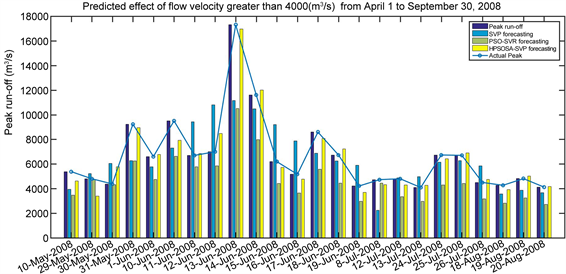Figure 6. Prediction results on runoff greater than 4000 (m3/s) from April 1 to September 30, 2008Table 4. Fitting and prediction results of three models during the 2009 flood season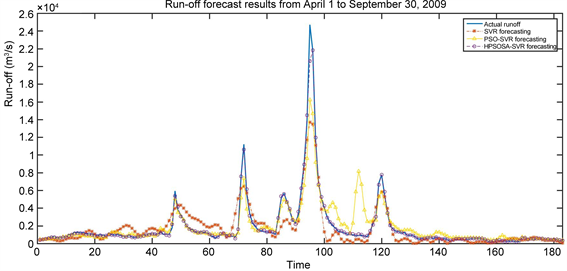Figure 7. Prediction results of runoff from April 1 to September 30, 2009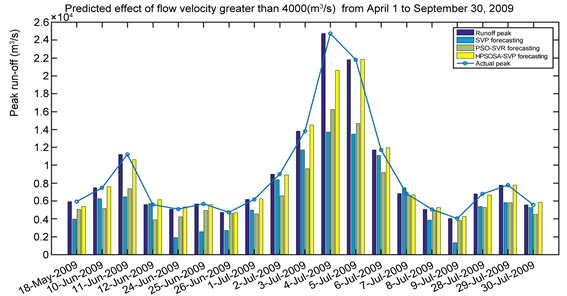Figure 8. Prediction results on runoff greater than 4000 (m3/s) from April 1 to September 30, 2009Table 5. Fitting and prediction results of three models during the 2010 flood season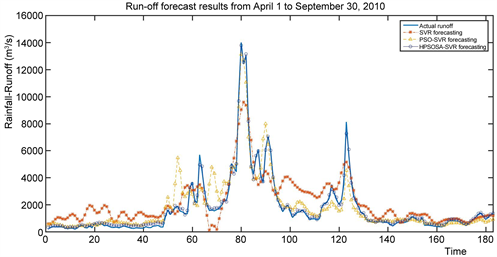Figure 9. Prediction results of runoff from April 1 to September 30, 2010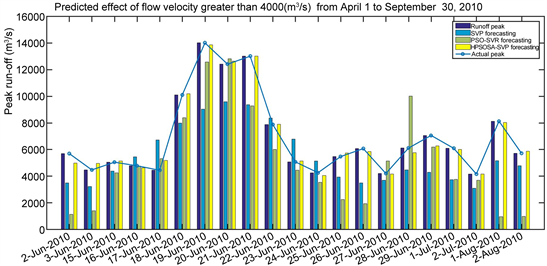Figure 10. Prediction results on runoff greater than 4000 (m3/s) from April 1 to September 30, 2010Table 6. Fitting and prediction results of three models during the 2010 flood season

4. 结语

1) HPSOSA-SVR算法能避免局部最优解，通过混合SA的接受准则和PSO随机接受原则，减少了局部搜索端点附近的震动偏离；根据新旧位置之间不同的适应度值，运用规则决定是接受新位置，还是重新计算另一个可信位置。这使得模型可以跳出局部最优解，并减少局部搜索终点附近的震动偏离。这些特性提高了解决方案的质量和收敛速度。

2) HPSOSA-SVR算法具有运算速度快和能在全局方向搜索最优解的优点，通过结合PSO运行机制、并行处理干扰机制以及SA解决搜索路径的特点，形成了一个良好的质量解决方案。

3) 根据本文的研究结果，我们可以得出以下结论：Sigmoid曲线核函数的SVR模型可以作为实际径流预测应用中的一种有效的核函数，而且最为适宜汛期径流SVR预测模型，预测结果精度高，稳定性好。

4) 采用Sigmoid核函数时，支持向量回归实现的是一种前向神经网络，但是由于使用SVM方法，网络隐含层节点数、连接权值都是在设计的过程中进化确定，而且SVR的理论决定了它最终求得的是全局最优值而不是局部最小值，也保证了它对于未知样本的良好泛化能力而不会出现过学习现象，以此可以解释Sigmoid曲线核函数的SVR模型最为适宜径流预测模型。

Evolving Support Vector Regression by Co-Evolution Algorithm for Runoff Forecasting Modeling Research and Application[J]. 计算机科学与应用, 2020, 10(03): 505-520. https://doi.org/10.12677/CSA.2020.103053

1. 1. Kisi, O., Shiri, J. and Tombul, M. (2013) Modeling Rainfall-Runoff Process Using Soft Computing Techniques. Computers & Geosciences, 51, 108-117. https://doi.org/10.1016/j.cageo.2012.07.001

2. 2. Rezaie-Balf, M., Zahmatkesh, Z. and Kim, S. (2017) Soft Computing Techniques for Rainfall-Runoff Simulation: Local Non-Parametric Paradigm vs. Model Classification Methods. Water Resources Management, 31, 3843-3865. https://doi.org/10.1007/s11269-017-1711-9

3. 3. Kumar, D., Sarthi, P.P. and Ranjan, P. (2016) Rainfall-Runoff Modeling Using Computational Intelligence Techniques. International Conference on Advances in Computing, Com-munications and Informatics, Jaipur, 21-24 September 2016, 800-806. https://doi.org/10.1109/ICACCI.2016.7732144

4. 4. Wu, J.S. and Xie, Y.S. (2019) Hybrid Support Vector Re-gression with Parallel Co-Evolution Algorithm Based on GA and PSO for Forecasting Monthly Rainfall. Journal of Software Engineering and Applications, 12, 524-539. https://doi.org/10.4236/jsea.2019.1212032

5. 5. Granata, F., Gargano, R. and De Marinis, G. (2016) Support Vector Regression for Rainfall-Runoff Modeling in Urban Drainage: A Comparison with The EPA’s Storm Water Management Mode. Water, 8, 69. https://doi.org/10.3390/w8030069

6. 6. Kan, G., Li, J., Zhang, X., et al. (2017) A New Hybrid Data-Driven Model for Event-Based Rainfall-Runoff Simulation. Neural Computing and Applications, 28, 2519-2534. https://doi.org/10.1007/s00521-016-2200-4

7. 7. Talei, A., Chua, L. H. and Quek, C. (2010) A Novel Application of a Neuro-Fuzzy Computational Technique in Event-Based Rainfall-Runoff Modeling. Expert Systems with Applica-tions, 37, 7456-7468. https://doi.org/10.1016/j.eswa.2010.04.015

8. 8. Wu, J.S., Jin, L. and Liu, M.Z. (2015) Evolving RBF Neural Networks for Rainfall Prediction Using Hybrid Particle Swarm Optimization and Genetic Algorithm. Neurocomputing, 148, 136-142. https://doi.org/10.1016/j.neucom.2012.10.043

9. 9. Wu, J.S. (2018) Co-Evolution Algorithm for Parameter Optimization of RBF Neural Networks for Rainfall-Runoff Forecasting. In: Huang, D.S., Bevilacqua, V., Premaratne, P. and Gupta, P., Eds., Intelligent Computing Theories and Application. ICIC, Lecture Notes in Computer Science, Springer, Cham, 195-206. https://doi.org/10.1007/978-3-319-95930-6_19

10. 10. Song, X., Kong, F., Zhan, C., et al. (2012) Hybrid Optimization Rainfall-Runoff Simulation Based on Xinanjiang Model and Artificial Neural Network. Journal of Hydrologic Engineering, 17, 1033-1041. https://doi.org/10.1061/(ASCE)HE.1943-5584.0000548

11. 11. Vapnik, V. (2013) The Nature of Statistical Learn-ing Theory. Springer Science & Business Media, Cham.

12. 12. 邓乃杨, 田英杰. 数据挖掘中的新方法-支持向量机[M]. 北京: 科学出版社, 2004.

13. 13. 朱波, 刘飞. 基于优化多核支持向量回归的制造过程均值偏移幅度估计[J]. 中国机械工程, 2014, 25(3): 630-635.

14. 14. 汪洪桥, 孙富春, 蔡艳宁, 等. 多核学习方法[J]. 自动化学报, 2010, 36(8): 1037-1050.

15. 15. Salat, R. and Salat, K. (2010) New Approach to Predicting Proconvulsant Activity with the Use of Support Vector Regression. Computers in Biology and Medicine, 42, 575-581. https://doi.org/10.1016/j.compbiomed.2012.02.001

16. 16. Kaytez, F., Taplamacioglu, M.C., Cam, E., et al. (2015) Forecasting Electricity Consumption: A Comparison of Regression Analysis, Neural Networks and Least Squares Support Vector Machines. International Journal of Electrical Power & Energy Systems, 67, 431-438. https://doi.org/10.1016/j.ijepes.2014.12.036

17. 17. 常群. 支持向量机的核方法及其模型选择[D]: [博士学位论文]. 哈尔滨: 哈尔滨工业大学, 2007.

18. 18. 罗林开. 支持向量机的核选择[D]: [博士学位论文]. 厦门: 厦门大学, 2007.

19. 19. 汪延华. 支持向量机模型选择研究[D]: [博士学位论文]. 北京: 北京交通大学, 2009.

20. 20. 廖杰, 王文圣, 李跃清, 等. 支持向量机及其在径流预测中的应用[J]. 四川大学学报(工程科学版), 2006, 38(6): 24-29.

21. 21. López, F.J.M., Puertas, S.M. and Arriaza, J.A.T. (2014) Training of Support Vector Machine with the Use of Multivariate Normalization. Applied Soft Computing, 24, 1105-1111. https://doi.org/10.1016/j.asoc.2014.08.020

22. 22. Güraksın, G.E., Haklı, H. and Uğuz, H. (2014) Support Vector Machines Classification Based on Particle Swarm Optimization for Bone Age Determination. Applied Soft Computing, 24, 597-602. https://doi.org/10.1016/j.asoc.2014.08.007

23. 23. Sheikhpour, R., Sarram, M.A. and Sheikhpour, R. (2016) Particle Swarm Optimization for Bandwidth Determination and Feature Selection of Kernel Density Estimation Based Classi-fiers in Diagnosis of Breast Cancer. Applied Soft Computing, 40, 113-131. https://doi.org/10.1016/j.asoc.2015.10.005

24. 24. Wu, J.S., Jin, L. and Liu, M. (2010) A Hybrid Support Vector Regression Approach for Rainfall Forecasting Using Particle Swarm Optimization and Projection Pursuit Technology. International Journal of Computational Intelligence and Applications, 9, 87-104. https://doi.org/10.1142/S1469026810002793

25. 25. Huang, C.L. and Wang, C.J. (2016) A GA-Based Feature Se-lection and Parameters Optimization for Support Vector Machines. Expert Systems with Applications, 31, 231-240. https://doi.org/10.1016/j.eswa.2005.09.024

26. 26. Oliveira, A.L.I., Braga, P.L., Lima, R.M.F., et al. (2010) GA-Based Method for Feature Selection and Parameters Optimization for Machine Learning Regression Applied to Software Effort Estimation. Information and Software Technology, 52, 1155-1166. https://doi.org/10.1016/j.infsof.2010.05.009

27. 27. Li, X., Wu, S., Li, X., et al. (2020)Particle Swarm Optimiza-tion-Support Vector Machine Model for Machinery Fault Diagnoses in High-Voltage Circuit Breakers. Chinese Journal of Mechanical Engineering, 33, 6. https://doi.org/10.1186/s10033-019-0428-5

28. 28. Yang, A., Li, W. and Yang, X. (2019) Short-Term Electricity Load Forecasting Based on Feature Selection and Least Squares Support Vector Machines. Knowledge-Based Systems, 163, 159-173. https://doi.org/10.1016/j.knosys.2018.08.027

29. 29. Poli, R., Kennedy, J. and Blackwell, T. (2007) Particle Swarm Optimization. Swarm Intelligence, 1, 33-57. https://doi.org/10.1007/s11721-007-0002-0

30. 30. Rini, D.P., Shamsuddin, S.M. and Yuhaniz, S.S. (2011) Particle Swarm Optimization: Technique, System and Challenges. International Journal of Computer Applications, 14, 19-26. https://doi.org/10.5120/1810-2331

31. 31. Dowsland, K.A. and Thompson, J. (2012) Simulated Annealing. In: Handbook of Natural Computing, Wiley, New York, 1623-1655. https://doi.org/10.1007/978-3-540-92910-9_49

32. 32. Van, Laarhoven, P.J.M. and Aarts, E.H.L. (1987) Simulated Annealing. In: Simulated Annealing: Theory and Applications, Springer, Dordrecht, 7-15. https://doi.org/10.1007/978-94-015-7744-1_2

33. 33. Mafarja, M.M. and Mirjalili, S. (2017) Hybrid Whale Optimization Algorithm with Simulated Annealing for Feature Selection. Neurocomputing, 260, 302-312. https://doi.org/10.1016/j.neucom.2017.04.053

34. 34. Assad, A. and Deep, K. (2018) A Hybrid Harmony Search and Simulated Annealing Algorithm for Continuous Optimization. Information Sciences, 450, 246-266. https://doi.org/10.1016/j.ins.2018.03.042